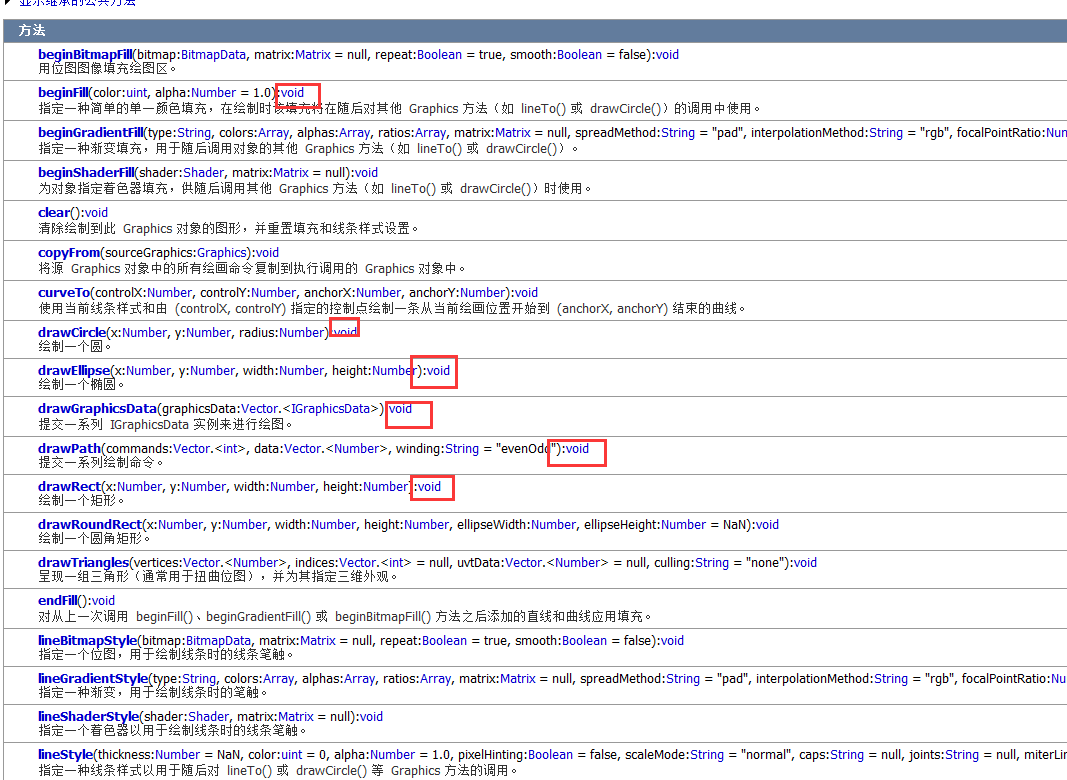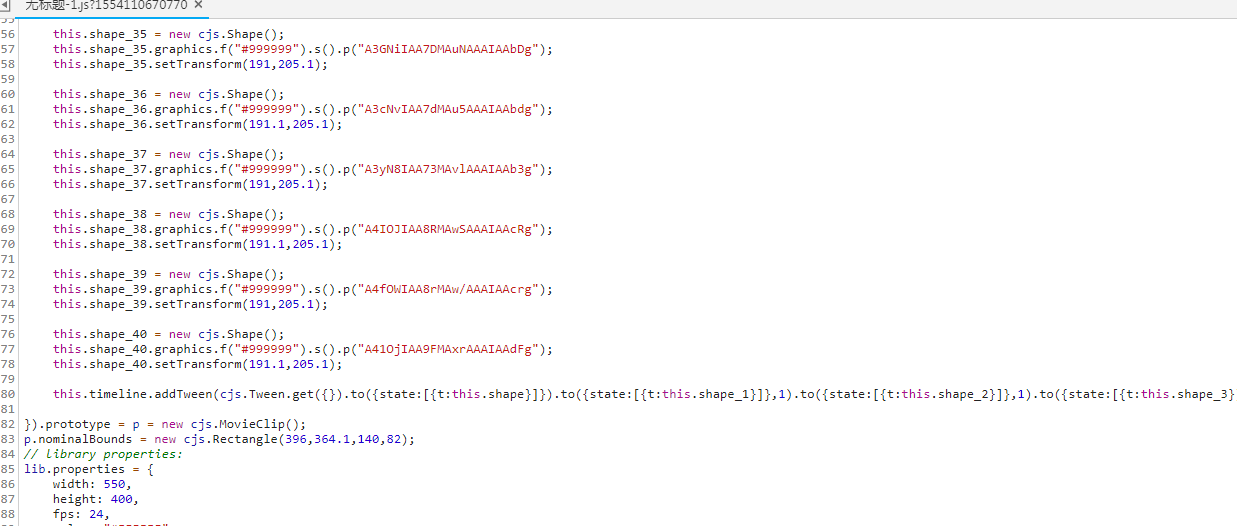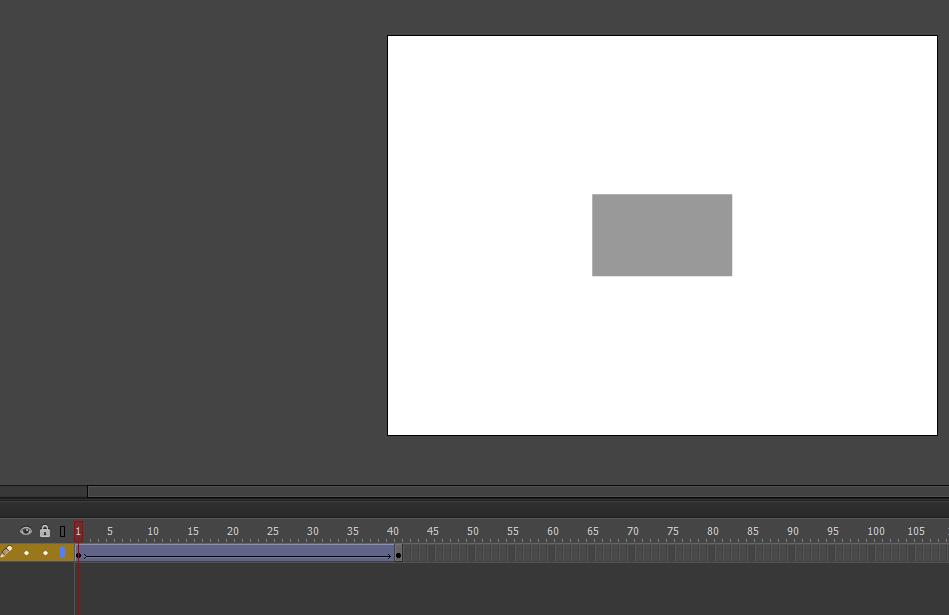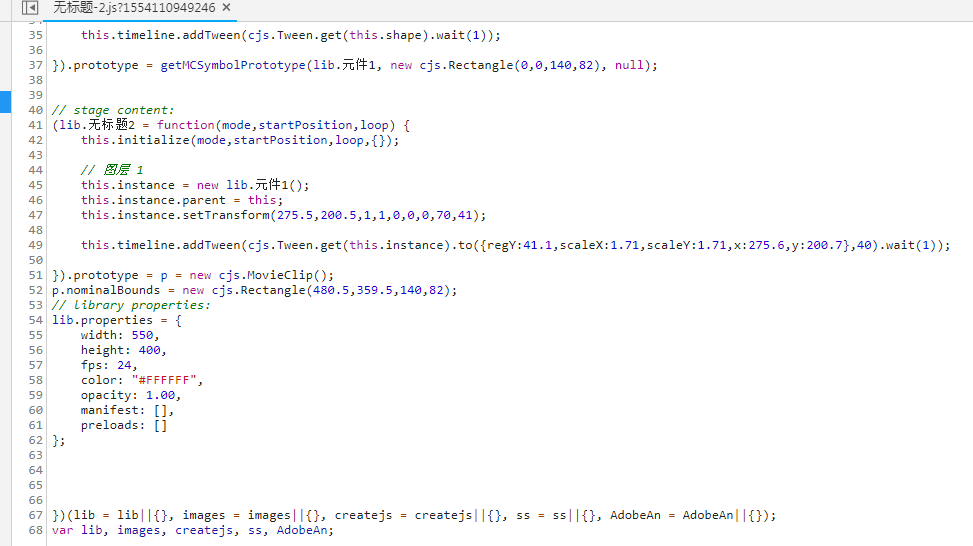## graphics.command的用法及AS3和Createjs矢量的区别

• 内容
• 评论
• 相关

```var point1 = new createjs.Point(50,50);
var point2 = new createjs.Point(200,200);
var shape = new createjs.Shape();

shape.graphics.clear()
shape.graphics.beginStroke("#ff0000").moveTo(point1.x,point1.y).lineTo(point2.x,point2.y).endStroke();
point2.x--;
if(point2.x < 0) point2.x = 200;
})```

```var point1 = new createjs.Point(50,50);
var point2 = new createjs.Point(200,200);
var shape = new createjs.Shape();
shape.graphics.beginStroke("#ff0000");
var moveCommand = shape.graphics.moveTo(0,0).command;
var lineCommand = shape.graphics.lineTo(0,0).command;

moveCommand.x = point1.x;
moveCommand.y = point1.y;
lineCommand.x = point2.x;
lineCommand.y = point2.y;
point2.x--;
if(point2.x < 0) point2.x = 200;
})```

（代码我写在fla里面，不会animate的可以复制上面代码放到自己的文件里）AS3由于用的是数据，所以几乎所有的api都不提供返回值，直接就把数据画到舞台上了，想要改变就只能clear。2：指令和指令之间是不可能有补间的（AS3因为是数据，他有内置的算法，所以可以自己演算补间），也就是说createjs的矢量和矢量时间是无法做形状补间的，那有人会问了，animateCC可以做形状补间啊，它是怎么做到的的呢？很简单，他直接发布成了逐帧动画。我来做一个例子，大家会animateCC的也可以随便做个例子。0条评论